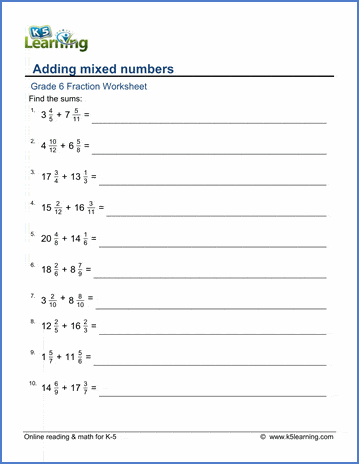## Fractions worksheet

These grade 6 fractions worksheets focus on adding and subtracting fractions and mixed numbers with unlike denominators.  All worksheets are pdf files and answer keys follow the questions on a separate page.

Adding unlike fractions 1/2 + 7/12=
Add unlike fractions (harder) 7/9 + 3/30=

Add fraction & mixed numbers 8/10 + 4 2/5 =
Add mixed numbers 5 4/5 + 6 2/3 =
Add mixed numbers (denominators to 30) 6 7/9 + 3 8/30 =
Add mixed numbers (denominators to 60) 8 9/16 + 4 8/50 =

## Fraction subtraction

Subtract unlike fractions 5/9 - 2/4 =
Subtract unlike fractions (denominators to 30) 9/24 - 1/5 =
Subtract unlike fractions (denominators to 60) 46/50 - 4/12 =

Subtract mixed numbers 7 1/4 - 4 1/2 =
Subtract mixed numbers (denominators to 30) 7 9/16 - 3 7/8 =
Subtract mixed numbers (denominators to 60) 7 51/52 - 4 3/4 =